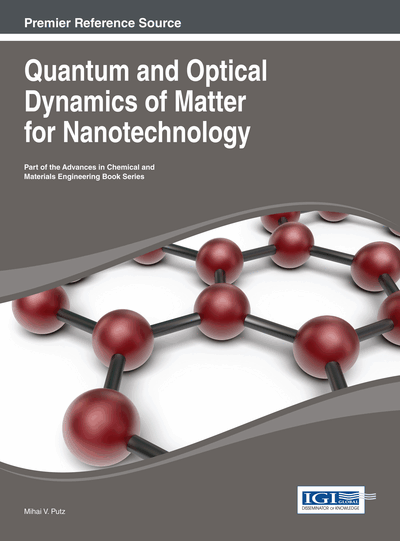# Classical and Quantum Theories of Dynamic X-Ray Diffraction

DOI: 10.4018/978-1-4666-4687-2.ch012

## Abstract

The main results of the dynamical theories of X-ray diffraction in their standard form of coupling of Maxwell equations with the Bloch waves on the one hand and the interaction of radiation-crystal in terms of perspective of quantum field on the other are unitarily presented.
Chapter Preview
Top

## 12.2. Semi-Classical Theory

As for the dynamic theory Laue, it will be expressed the electronic density in any point of the crystal as Fourier sum over the reciprocal space associated (Batterman & Cole 1964):

(12.1) whereis the volume unit,is the vector of the reciprocal space expressed based on the Miller indices and the vectors of the its main directions. The reciprocal relation (12.1) will unfold of the structure factor as the Fourier continuous transform of the punctual electronic density over the unit cell:

(12.2)

Need to further consider how is affected the dielectric constant of the medium of the electronic density correlated with the structure factor (12.1), because it links the induction and the electric intensity in the Maxwell equations, allowing their uniform solution, as we shall see.

For an environment with intrinsic polarizationthere is the bond:

(12.3) whereis the dielectric constant, andis the electric permittivity of the vacuum.

If we consider an external sinusoidal electric field of pulsation much higher than its own pulsation of oscillation of the electrons on their intrinsic energetic levels,, the second equation of Newton, the equation of the restoration of the movement of oscillation in the external field, will give the amplitude this movement:

(12.4) where,is the amplitude of the external field applied, and e andare the charge and respectively the mass of the electron.

## Complete Chapter List

Search this Book:
Reset